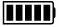ECE4253 Digital Communications Department of Electrical and Computer Engineering - University of New Brunswick, Fredericton, NB, Canada

# Galois Field GF(2) Calculator

 Model C-172 POLYNOMIAL CALCULATORA:

B:

 A + B A - B A × B A / B

Discussion  Polynomials  MATLAB

``` 11000101
---------- = 11111, Remainder = 11
1010```

## Detailed Calculation

 ``` 11111 ---------- 1010 ) 11000101 1010 ---- 1100101 1010 ---- 110101 1010 ---- 11101 1010 ---- 1001 1010 ---- 0011```

# MATLAB Example

Calculations in GF(2) are performed by MATLAB functions found within the Communications Toolbox.

>> a = gf( [1 1 0 0 0 1 0 1] );

>> b = gf( [1 0 1 0] );

>> [q,r] = deconv(a,b) % deconvolution is equivalent to division

q = GF(2) array.

Array elements =

1      1      1      1      1

r = GF(2) array.

Array elements =

0      0      1      1

 2021-09-22 09:30:03 ADT Last Updated: 2010-04-29 Richard Tervo [ tervo@unb.ca ]